# Number Systems Binary Decimal Hexadecimal Bits Bytes and

• Slides: 25Number Systems Binary Decimal HexadecimalBits, Bytes, and Words A bit is a single binary digit (a 1 or 0). p A byte is 8 bits p A word is 32 bits or 4 bytes p Long word = 8 bytes = 64 bits p Quad word = 16 bytes = 128 bits p Programming languages use these standard number of bits when organizing data storage and access. p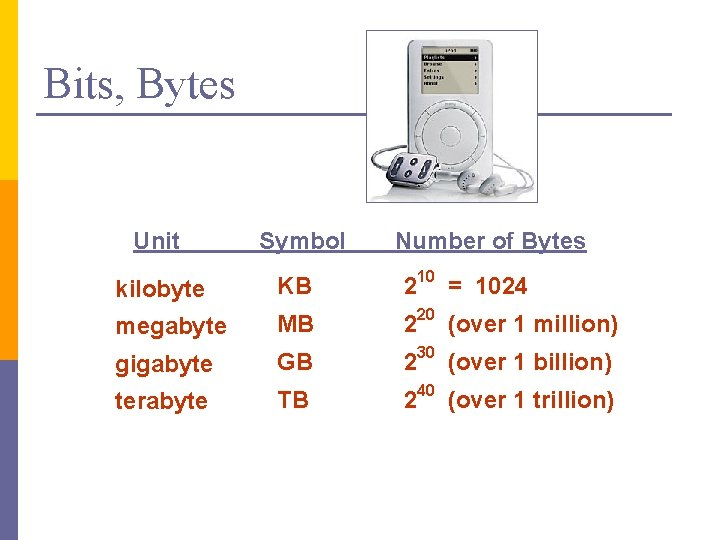Bits, Bytes Unit Symbol Number of Bytes kilobyte KB 210 = 1024 megabyte MB 220 (over 1 million) gigabyte GB 230 (over 1 billion) terabyte TB 240 (over 1 trillion)Bit Permutations p 1 bit(only 1 light bulb ): Option: 1 Option: 2 0 1Bit Permutations - 2 bit Option: 0 0 Option: 1 0 0 Option: 3 Option: 2 1 1 0 1 1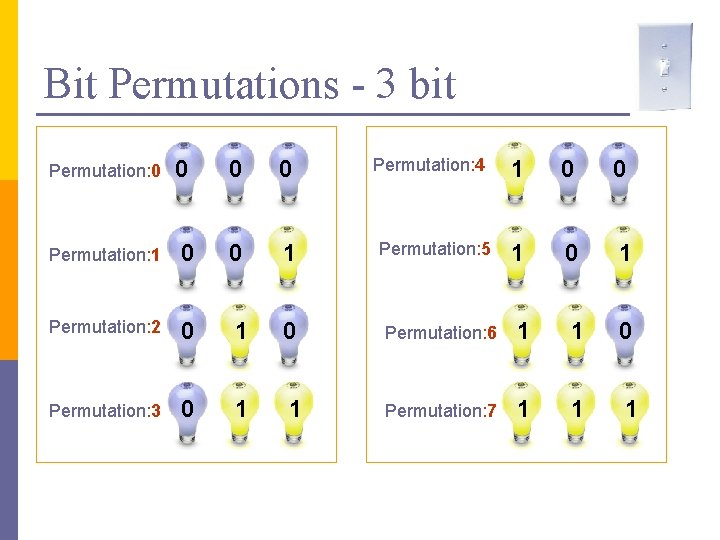Bit Permutations - 3 bit 0 0 0 Permutation: 1 0 0 1 Permutation: 2 0 1 0 Permutation: 3 0 1 1 Permutation: 0 Permutation: 4 1 0 0 1 Permutation: 6 1 1 0 Permutation: 7 1 1 1 Permutation: 5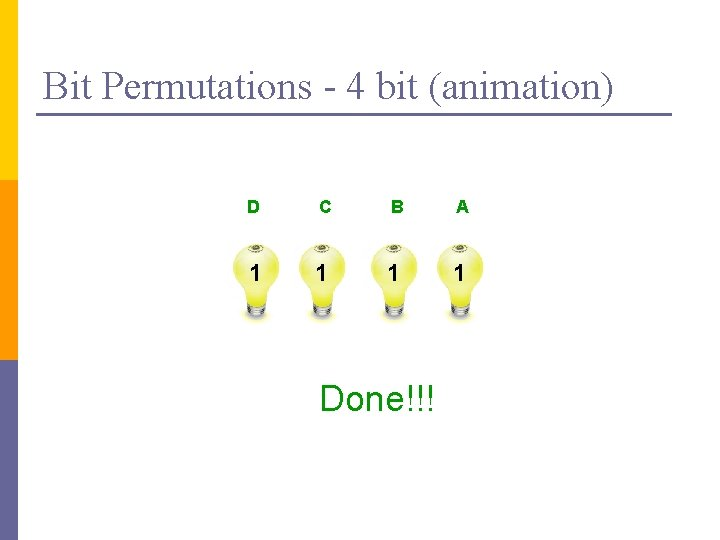Bit Permutations - 4 bit (animation) D C B A 1 0 0 1 010 0 10 Done!!!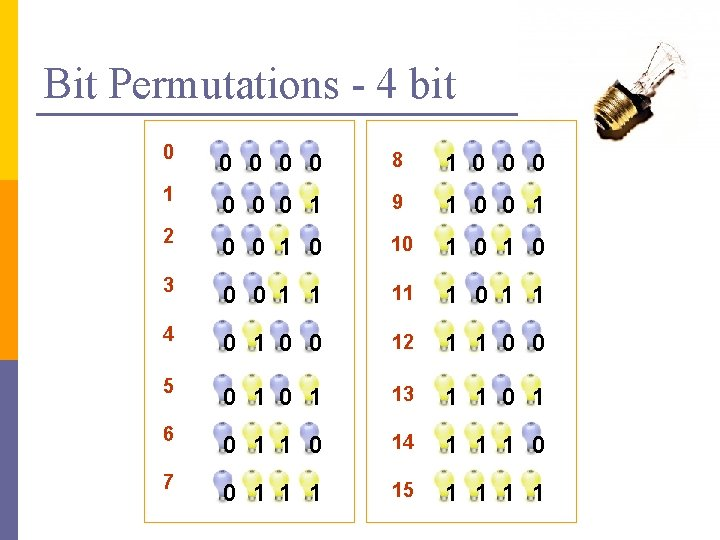Bit Permutations - 4 bit 0 0 0 8 1 0 0 0 1 9 1 0 0 1 2 0 0 10 1 0 3 0 0 1 1 11 1 0 1 1 4 0 1 0 0 12 1 1 0 0 5 0 1 13 1 1 0 1 6 0 1 1 0 14 1 1 1 0 7 0 1 15 1 1Bit Permutations 1 bit 0 1 2 bits 00 01 10 11 3 bits 000 001 010 011 100 101 110 111 4 bits 0000 1000 0001 1001 0010 1010 0011 1011 0100 1100 0101 1101 0110 1110 0111 1111 Each additional bit doubles the number of possible permutationsNumber Systems The on and off states of the capacitors in RAM can be thought of as the values 1 and 0, respectively. p Therefore, thinking about how information is stored in RAM requires knowledge of the binary (base 2) number system. p Let’s review the decimal (base 10) number system first. p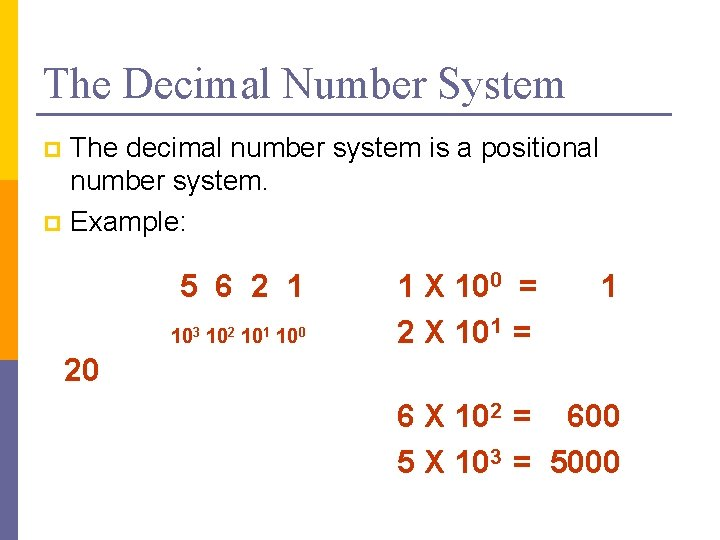The Decimal Number System The decimal number system is a positional number system. p Example: p 5 6 2 1 103 102 101 100 1 X 100 = 2 X 101 = 1 20 6 X 102 = 600 5 X 103 = 5000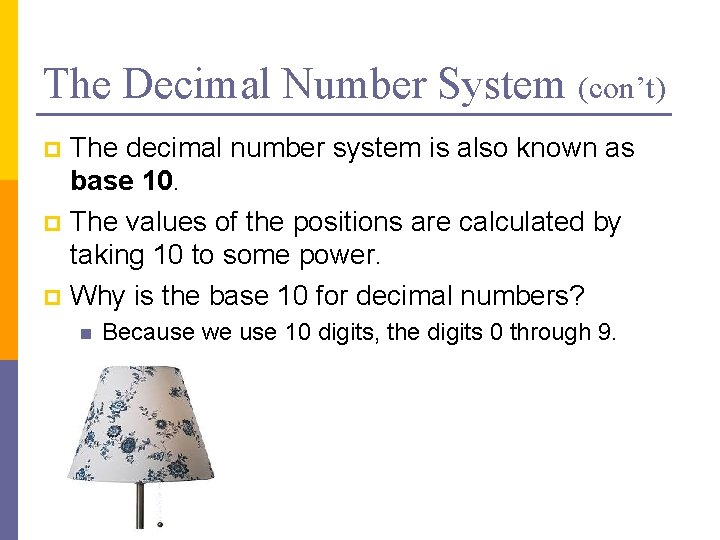The Decimal Number System (con’t) The decimal number system is also known as base 10. p The values of the positions are calculated by taking 10 to some power. p Why is the base 10 for decimal numbers? p n Because we use 10 digits, the digits 0 through 9.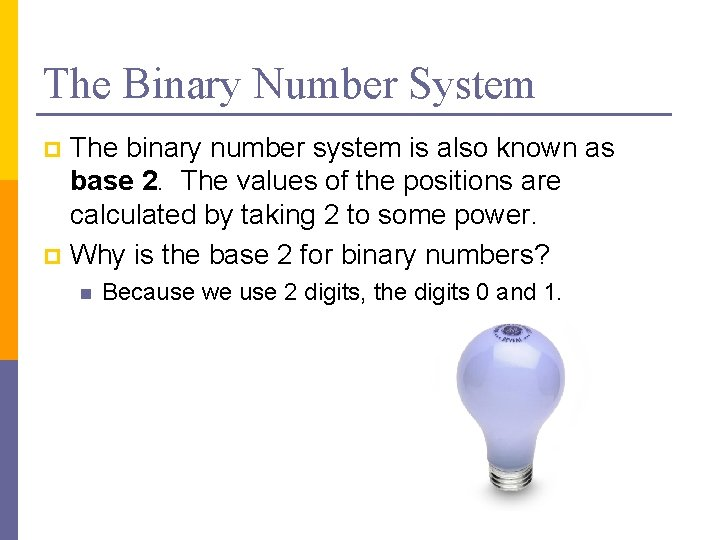The Binary Number System The binary number system is also known as base 2. The values of the positions are calculated by taking 2 to some power. p Why is the base 2 for binary numbers? p n Because we use 2 digits, the digits 0 and 1.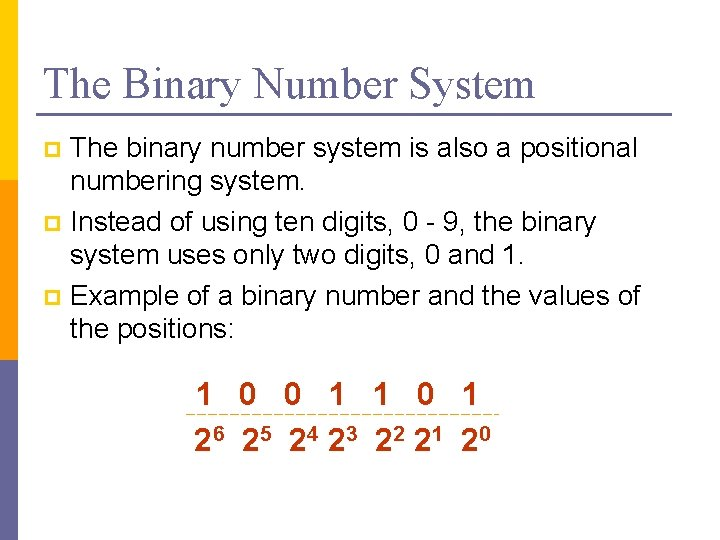The Binary Number System The binary number system is also a positional numbering system. p Instead of using ten digits, 0 - 9, the binary system uses only two digits, 0 and 1. p Example of a binary number and the values of the positions: p 1 0 0 1 1 0 1 2 6 2 5 2 4 23 2 2 21 2 0Converting from Binary to Decimal 1 0 0 1 1 0 1 2 6 2 5 24 2 3 2 2 2 1 20 20 = 1 21 = 2 22 = 4 23 = 8 24 = 16 25 = 32 26 = 64 1 X 20 = 1 0 X 21 = 0 1 X 22 = 4 1 X 23 = 8 0 X 24 = 0 0 X 25 = 0 1 X 26 = 64 7710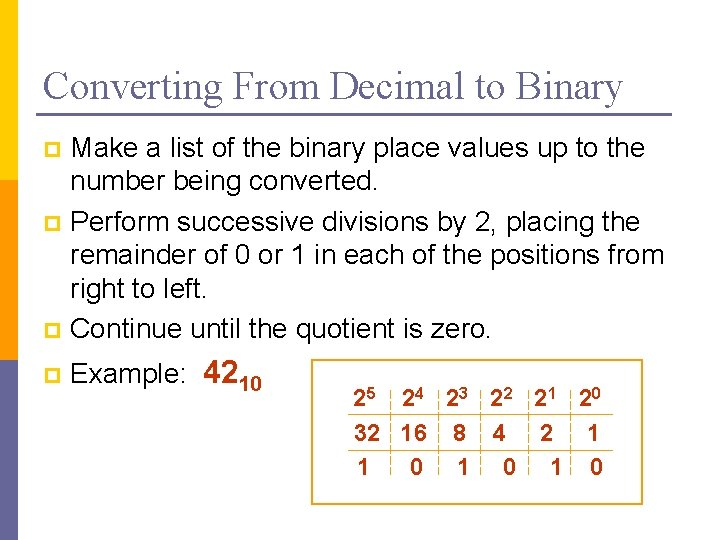Converting From Decimal to Binary Make a list of the binary place values up to the number being converted. p Perform successive divisions by 2, placing the remainder of 0 or 1 in each of the positions from right to left. p Continue until the quotient is zero. p p Example: 4210 25 24 23 22 21 20 32 16 8 4 2 1 1 0 1 0Adding Binary carries overflow 11111 111011 +100111 1100010 carries overflow 1 1 11001 +01101 100110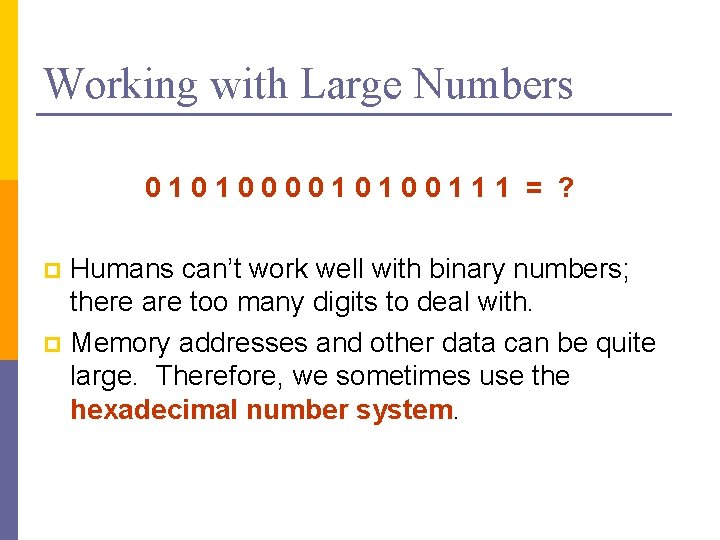Working with Large Numbers 0101000010100111 = ? Humans can’t work well with binary numbers; there are too many digits to deal with. p Memory addresses and other data can be quite large. Therefore, we sometimes use the hexadecimal number system. pThe Hexadecimal Number System p p The hexadecimal number system is also known as base 16. The values of the positions are calculated by taking 16 to some power. Why is the base 16 for hexadecimal numbers ? n Because we use 16 symbols, the digits 0 through 9 and the letters A through F.The Hexadecimal Number System Binary Decimal 0 0 0 1010 10 A 1 2 3 4 5 6 7 8 9 1011 1100 1101 1110 1111 11 12 13 14 15 B C D E F 1 10 11 100 101 110 111 1000 1001 Hexadecimal Binary Decimal Hexadecimal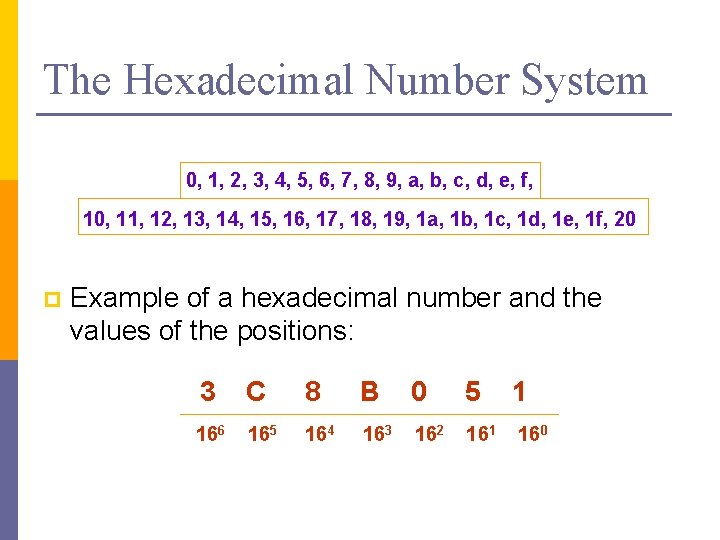The Hexadecimal Number System 0, 1, 2, 3, 4, 5, 6, 7, 8, 9, a, b, c, d, e, f, 10, 11, 12, 13, 14, 15, 16, 17, 18, 19, 1 a, 1 b, 1 c, 1 d, 1 e, 1 f, 20 p Example of a hexadecimal number and the values of the positions: 3 C 8 B 0 5 166 165 164 163 162 161 1 160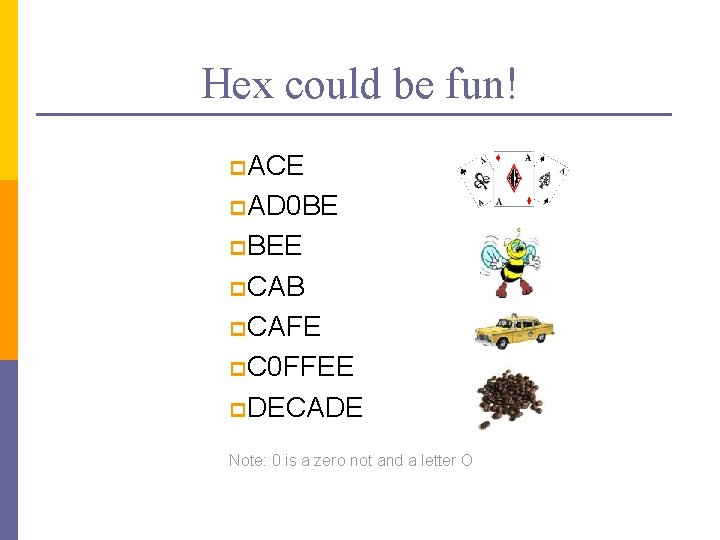Hex could be fun! p ACE p AD 0 BE p BEE p CAB p CAFE p C 0 FFEE p DECADE Note: 0 is a zero not and a letter O